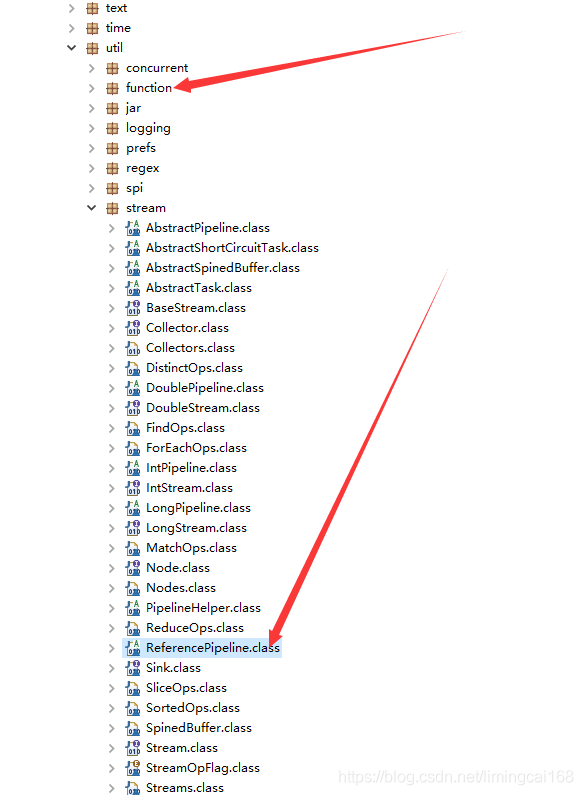• 如果您觉得本站非常有看点，那么赶紧使用Ctrl+D 收藏吧

# JAVA8 Stream 和Lambda表达式使用

Lambda也叫函数式编程   包括此方法都是（Function<? super R, ? extends V> after）

1 首生 Lambd表达式简化了方法的定义，匿名的方法直接实现逻辑  使用方式  （x, y) -> x + y; 返回x+y之和，有参数的匿名 ，无参方法，  () -> { System.out.println(“Hello Lambda!”); } ,不需要写方法单独去实现。

``````    //中国的IP，记录下来，跳过此IP去分配内网IP
final String[] cn_ip_str = {""};
list_ip.stream().forEach(nodeip -> {
try {
Country country = response.getCountry();
CountryModel model = new CountryModel();
model.setName(country.getNames().get("zh-CN"));
model.setIsoCode(country.getIsoCode());
model.setCountryIp(nodeip);
if (country.getIsoCode().equals("CN")) {
cn_ip_str = nodeip;
}
System.out.println(model.getName() + ", EN: " + model.getIsoCode() + " IP: " + model.getCountryIp());
} catch (IOException e) {
e.printStackTrace();
} catch (GeoIp2Exception e) {
e.printStackTrace();
}
});``````

PS  类名.方法名  =  ::  ,   Arraylist::new  , ClassName:getName

例1   list.forEach(o -> {System.out.println(o);}); //forEach函数实现内部迭代  System.out::println 效果一样

`````` list.stream().filter(smap -> null != smap.get("ip") && !"".equals(smap.get("ip"))).forEach(imp -> {
});
``````

2  结全Stream  的流式处理，对于list的遍历更加节省代码量，简洁高效

Java8为集合类引入了另一个重要概念：流（stream）。一个流通常以一个集合类实例为其数据源，然后在其上定义各种操作。流的API设计使用了管道（pipelines）模式。对流的一次操作会返回另一个流。如同IO的API或者StringBuffer的append方法那样，从而多个不同的操作可以在一个语句里串起来。看下面的例子：

List<Shape> shapes = …
shapes.stream()
.filter(s -> s.getColor() == BLUE)
.forEach(s -> s.setColor(RED));

shapes.parallelStream(); // 或shapes.stream().parallel()

PS 下面是典型的大数据处理方法，Filter-Map-Reduce：

//给出一个String类型的数组，找出其中所有不重复的素数
public void distinctPrimary(String… numbers) {
List<String> l = Arrays.asList(numbers);
List<Integer> r = l.stream()
.map(e -> new Integer(e))
.filter(e -> Primes.isPrime(e))
.distinct()  //去重
.collect(Collectors.toList());   //（reduce）代入
System.out.println(“distinctPrimary result is: ” + r);
}

reduce方法用来产生单一的一个最终结果  .distinct().reduce(0, (x,y) -> x+y); // equivalent to .sum()。

int sum = l.stream()
.map(e -> new Integer(e))
.filter(e -> Primes.isPrime(e))
.distinct()
.reduce(0, (x,y) -> x+y); // equivalent to .sum()

Integer::parseInt //静态方法引用
System.out::print //实例方法引用
Person::new       //构造器引用

PS  下面求和的简洁方法，聚合函数都可以实现

public void distinctPrimarySum(String… numbers) {
List<String> l = Arrays.asList(numbers);
int sum = l.stream().map(Integer::new).filter(Primes::isPrime).distinct().sum();
System.out.println(“distinctPrimarySum result is: ” + sum);
}

Stream.generate(Math::random).limit(5).forEach(System.out::println);

jdk1.8，  rt.jar包下面的函数式编程和流式处理包  流式处理是用的管道